## 負載電流計算

2016/8/16 下午 10:20★ 非常重要！配電系統中，包括線纜、開關和變壓器 … 等之選用，都跟負載電流有關 ★

S = V * I = P / pf = P / cosθ

I_(1∮)=S/V_P =P/〖pf×V〗_P =P/cos⁡〖θ×V_P 〗

I_(3∮)=S/〖√3×V〗_L =P/〖√3×pf×V〗_L =P/〖√3×cos⁡θ×V〗_L

Δ 接三相電源，線電壓VL = 相電壓 VP Ｙ接三相電源，線電壓VL = √3 * 相電壓 VP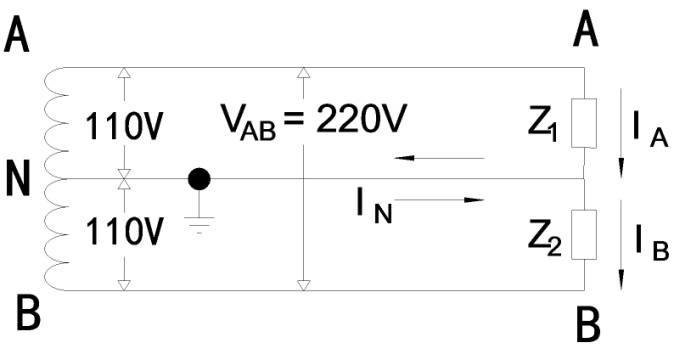IA = （3 KW / 0.8）/ 0.11 KV = 34.09 A（由A經Z1流向N）
IB = （7 KW / 0.8）/ 0.11 KV = 79.55 A（由B經Z2流向N）

IA = IB + IN
IN = IA - IB = 79.55 - 34.09 = 45.46 A ≠ 0 A（負載不平衡，因IA > IB，故IN 流動方向為由左而右）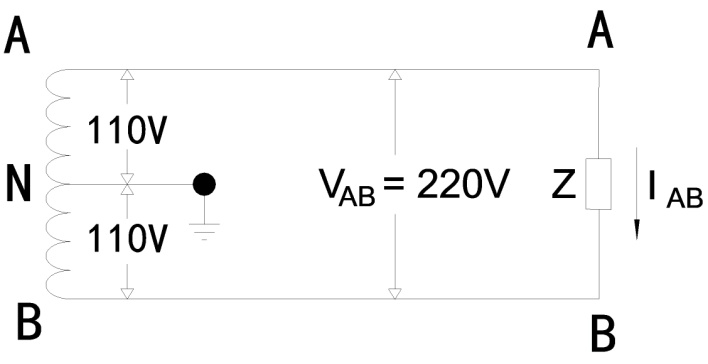IAB = （10 KW / 0.8）/ 0.22 KV = 56.82 A（由A經Z流向B）
IN = 0 A（負載平衡）

Δ 接三相平衡負載Δ 接三相電源， 線電壓VL = 相電壓 VP

IA = IAB + IAC，IB = IBA + IBC，IC = ICA + ICB　（向量和）
IL = √3 * IP （有效值）P1∮ = VP * IP * cosθ

IBC = P1∮Z2 /（cosθ2 * VBC） IBA = P1∮Z1 /（cosθ1 * VBA）
ICA = P1∮Z3 /（cosθ3 * VCA） ICB = P1∮Z2 /（cosθ2 * VCB）

P3∮ = 3 * VP * IP * cosθ = 3 * VP * IP * cosθ = 3 * VL *（IL / √3）* cosθ = √3 * VL * IL* cosθ

Δ 接三相負載線電流IL = （P3∮ / cosθ）/ （√3 * VL）= S3∮ /（√3 * VL）

Y 接三相平衡負載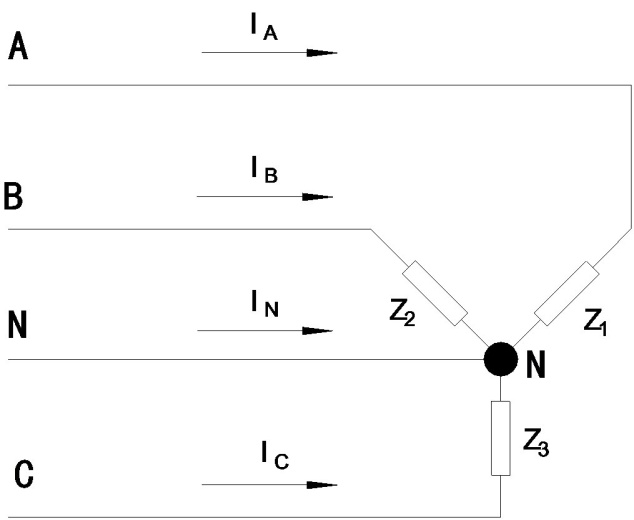Y 接三相電源，線電壓VL = √3 * 相電壓VP，線電流 IL =相電流IP

IBN = P1∮Z2 /（cosθ2 * VBC）
ICＮ = P1∮Z3 /（cosθ3 * VCA）

P3∮ = P1∮Z1 + P1∮Z2 + P1∮Z3 = 3 * P1∮

= 3 * P1∮ = 3 *（VL / √3） * IL* cosθ = √3 * VL * IL* cosθ

Y 接三相負載線電流IL = （P3∮ / cosθ）/ （√3 * VL）= S3∮ /（√3 * VL）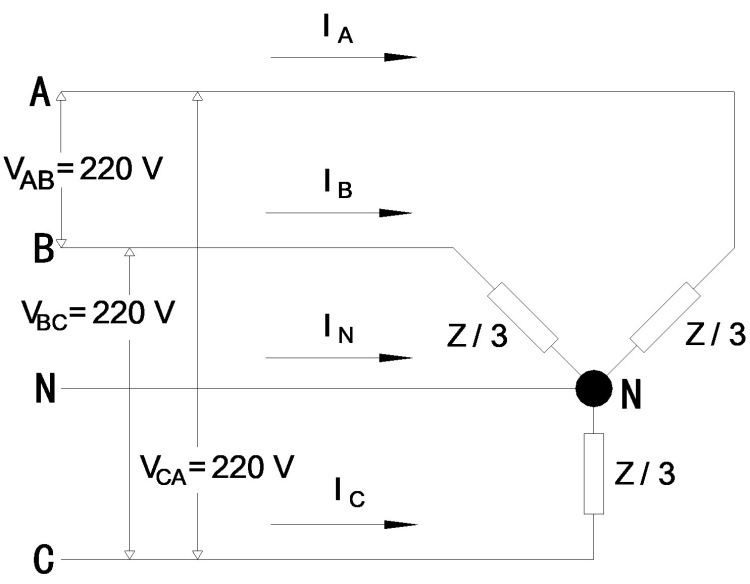IL =（10 KW / 0.8）/（√3 * 0.38 KV）= 18.99 A（由A、B、C分別經Z / 3流向N）
IA = IB = IC = IL

IAN = IA = 18.99A，IBN = IB = 18.99A，ICN = IC = 18.99A

IAN + IBN + ICN + IN = 0
IN = - IAN - IBN - ICN = - 18.99∠0o - 18.99∠120o - 18.99∠240o
= -（18.99cos0o + j 18.99sin0o）-（18.99cos120o + j 18.99sin120o）-（18.99cos240o + j 18.99sin240o）
= -（18.99 + j 0）-（-9.495 + j 16.45）-（-9.495 + j -16.45）
=（-18.99 - j 0）+（9.495 - j 16.45）+（9.495 + j 16.45）
=（- 18.99 + 9.495 + 9.495）+ j（-16.45 + 16.45）
= 0 + j 0 = 0 A（負載平衡，三相設備）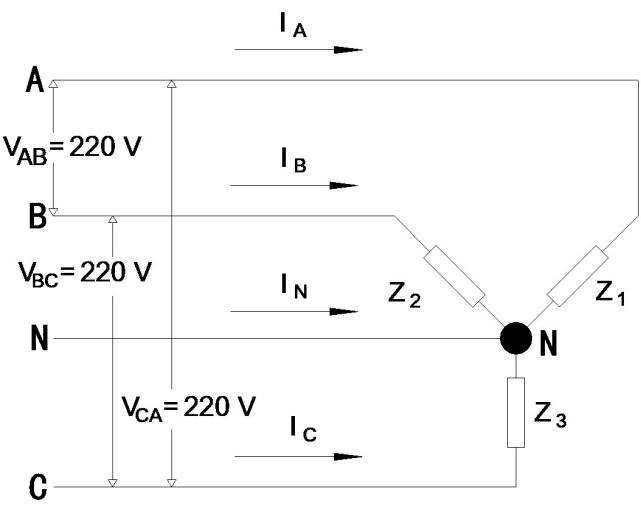IAN = （2 KW / 0.6）/（0.22 KV） = 15.14 A（由A經Z1流向N）
IBN = （3 KW / 0.7）/（0.22 KV） = 19.5 A（由B經Z2流向N）
ICN = （5 KW / 0.8）/（0.22 KV） = 28.41 A（由C經Z3流向N）

IA = IAN = 15.15 A，IB = IBN= 18.49 A，IC=ICN = 28.41 A

IAN + IBN + ICN + IN = 0

IN = - IAN - IBN - ICN = - 15.15∠0o - 19.48∠120o - 28.41∠240o
= -（15.15 cos0o + j 15.15 * sin0o）-（19.48 cos120o + j 19.48 sin120o）-（28.41 cos240o + j 28.41 sin240o）
= -（15.15 + j 0）-（-9.74 + j 16.87）-（-14.21 - j 24.6）
=（-15.15 - j 0）+（9.74 - j 16.87）+（14.21 + j 24.6）
=（- 15.15 + 9.74 +14.21）+ j（-16.87 + 24.6）
= 8.8 + j 7.73 = 11.71 ∠41.3o （負載不平衡，發生在單相設備）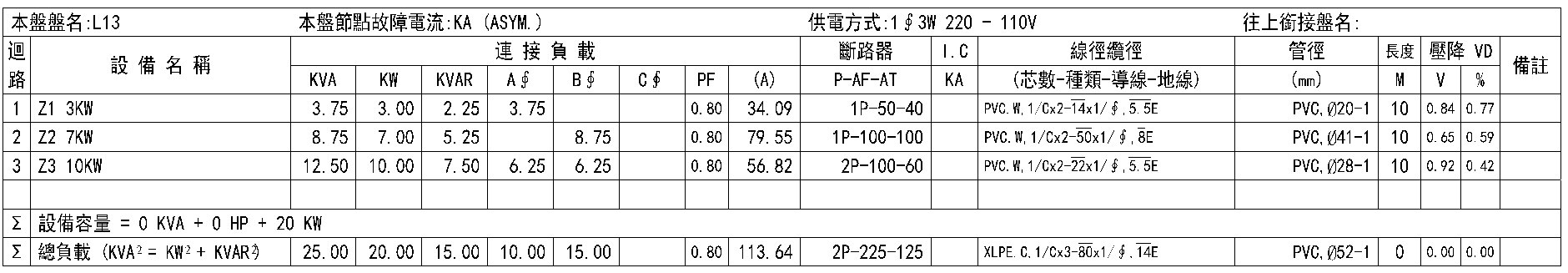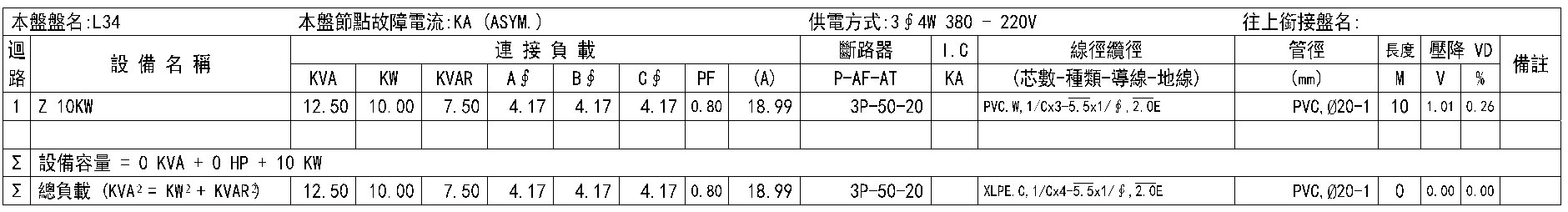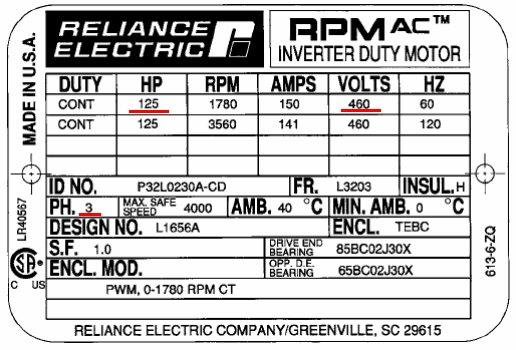I_(3∮)=P/〖√3×pf×η×V〗_L

pf=(HP×0.75)/(√3×I_(3∮)×V_L )